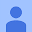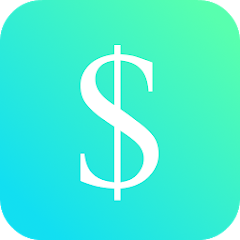3.9
1.25K reviews
100K+Everyone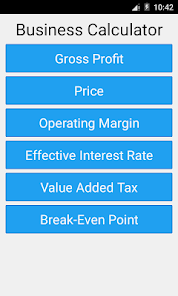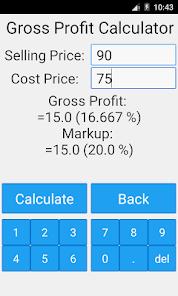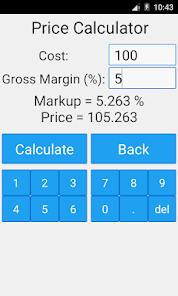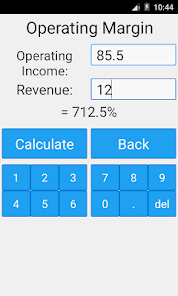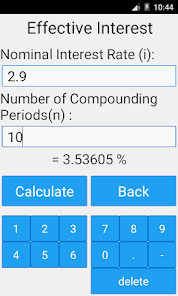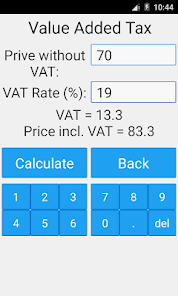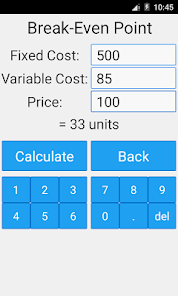This app consists of a Gross Profit Calculator, Price Calculator, Operating Margin Calculator, Effective Interest Rate Calculator, Value Added Tax Calculator and a Break-Even Point Calculator.

Gross Profit Calculator: You are able to calculate the gross profit (absolute value and percentage) and markup (absolute value and percentage). Just enter the cost price and selling price of the product.

Price Calculator: You are able to calculate the price and markup. Just enter the cost and gross margin.

Operating Margin Calculator: You are able to calculate the operating margin. Just enter the operating income and revenue.

Effective Interest Rate Calculator: You are able to calculate the effective interest rate. Just enter the nominal interest rate and the number of compounding periods per year.

Value Added Tax: You are able to calculate the VAT (value added tax) and the price incl. VAT. Just enter the price without VAT and the VAT rate of your country.

Break-Even Point: You are able to calculate the break-even point. Just enter fixed cost, variable cost and price of a unit.
Updated on
Apr 18, 2016

## Data safety

Safety starts with understanding how developers collect and share your data. Data privacy and security practices may vary based on your use, region, and age. The developer provided this information and may update it over time.No data shared with third partiesNo data collected

## Ratings and reviews

3.8
1.22K reviews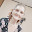Tracie Ann Howard Watkins
December 19, 2022
Fairly easy to use. Pretty straight forward inputting of information to achieve the correct calculations needed to determine all different aspects of business type calculations. In response to a review below... It's designed for business calculations not standard functions; so unless it's to be marketed as an all in one calculator, what's the point of having standard functions on there? It's not needed. I agree the separation of the keyboard is a little bit odd but it all still works!